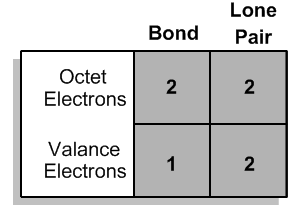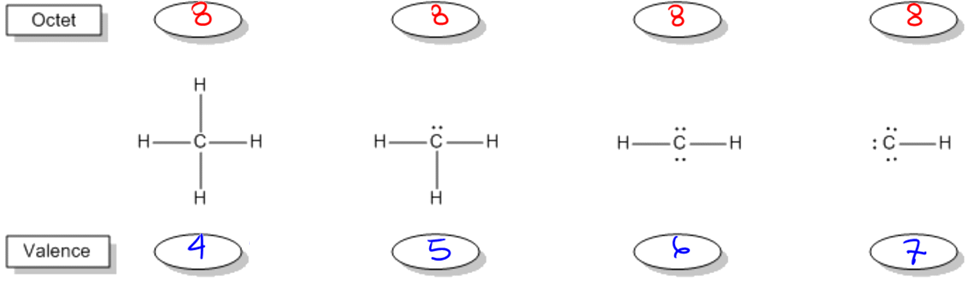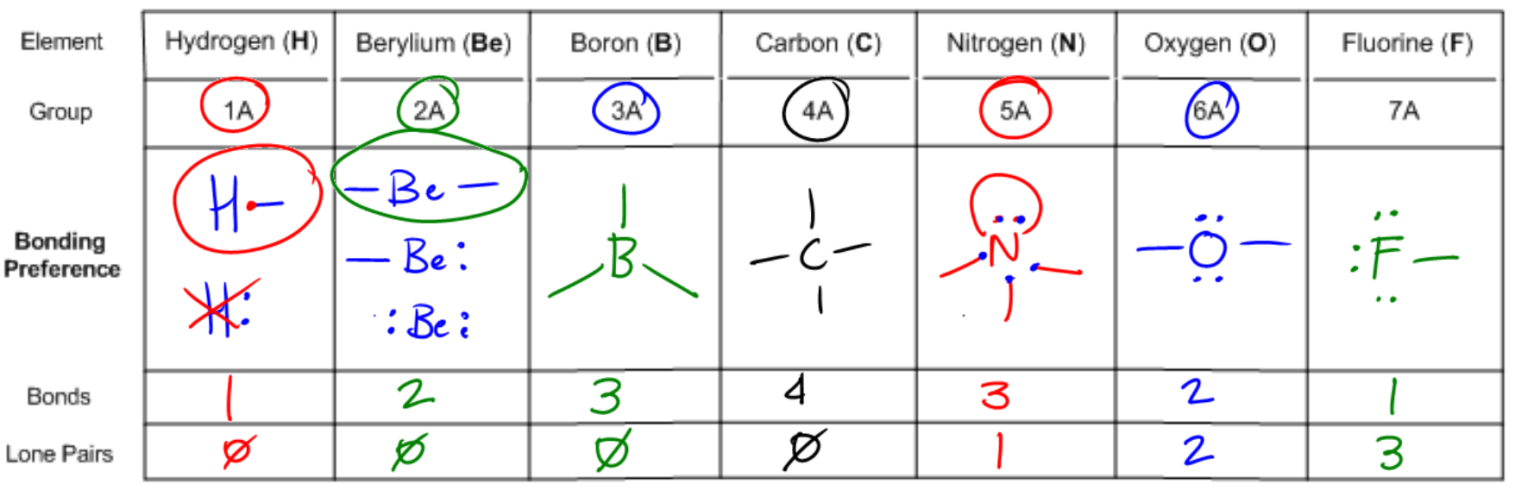Clutch Prep is now a part of Pearson
Ch. 1 - A Review of General ChemistryWorksheetSee all chapters

# Bonding Preferences

See all sections
Sections
Intro to Organic Chemistry
Atomic Structure
Wave Function
Molecular Orbitals
Sigma and Pi Bonds
Octet Rule
Bonding Preferences
Formal Charges
Skeletal Structure
Lewis Structure
Condensed Structural Formula
Degrees of Unsaturation
Constitutional Isomers
Resonance Structures
Hybridization
Molecular Geometry
Electronegativity
Isomer
Polar Vs. Nonpolar
Covalent Bond
Polar Bond

Just because an atom satisfies its octet doesn’t mean that it is stable. We also have to consider valence electrons. Sounds familiar? Let’s look into what those are.

###### Valence Electrons

Concept #1: What is a valence electron?

Transcript

So now let's talk about probably one of the most helpful topics in this entire chapter and that's the topic of bonding preferences. Now, this is something that I remember when I was in undergrad and I was taking orgo, I was really confused about because my professor a lot of times would just assume that I understood how atoms worked and how atoms bonded. I remember my professor would be like, “Oh, nitrogen forms three bonds and oxygen with a positive charge forms three bonds,” and I thought I was supposed to memorize all this stuff. It was really confusing.
It turns out that there's really simple logic that we can use to figure out exactly how many bonds every atom wants to have. Once you have this down, you're never going to forget it. Let's go ahead and move just right along.
Bonding preferences are based on the concept of octet electrons, but also based on another type of electron, so let's talk about it. It turns out that there are actual several ways to combine octet electrons in order to satisfy the octet rule for an atom.
Valence electrons, so this is a new word, valence electrons are the names that we give to the octet electrons that the atom actually owns. Remember that we said that atoms can choose to share electrons in bonds or they can choose to have electrons as lone pairs, both of them are going to count the same in terms of octet electrons because they're surrounding their part of the shell. But, they're actually going to count differently in terms of the valence count, so let's talk about that.
The number of the valence electrons is going to determine which of the octets that you could make. If there's several versions of octets, it's going to determine which of the octets is the most stable. This is the really basic rule. What we're going to say is that an atom is going to own every lone electron that it has and it's going to own only one electron for every bond that it has.
A really nice easy way to say this is that it's going to own every dot, one electron for every dot, and it's also going to own one electron for every stick. So I'm just going to say dot equals one and also stick equals one. Okay, that's just another way to say it. Alright.Every dot = 1 valence electron, and every stick = 1 valence electron. (Octet is different, see above.)

###### Valence Electrons and Stability

Concept #2: What is the difference between valence and octet electrons?

Transcript

So let's go ahead and do this kind of worked example and you guys can help me figure out the differences in stability for these.
The first thing I want you guys to do, we just talked about the octet rule, so what I want you guys to do is to figure out how many octet electrons each of these carbons would have. So go ahead and start off from the left, right there, and go ahead and pick out how many octet electrons does it have? 8. Okay, so let's write that in.
Now, what I want you guys to do is look at the next one and say, “Okay, how many octet electrons does that one have?” So let's move to the right and you're going to find there is that you also have 8. It's different though. It looks a little different, but I have two from the lone pair and two from each of these bonds, so it's still 8.
Now, we're going to keep going and what you're going to find is that all of these fulfill the octet rule. Okay. They all give carbon 8 electrons. Does that make sense so far? Even though they look radically different, but they still follow the octet rule.
So now here's my next question. Do you think that these are all equally stable? Do you think that they could all exist in the same way? It turns out, no. Definitely not. It turns out one of these is way more stable than all the other ones and the reason has to do with valence electrons. The way that we count valance electrons is remember we count dots and sticks, so what I want you guys to do now is count what are the valence electrons for all of these.
Let's start off with the one on the left. How many sticks does it have? 4. How many dots does it have? 0. So it would have 4 valence electrons.
Alright, the next one. How many dots does it have? 2. How many sticks does it have? 3. 2 + 3 is 5.
And you could keep going. What you're going to find is that this one has 6 valence electrons and this one has 7 valence electrons.
Alright, so what we find is that the octet rule is being satisfied with all of these, but they have very different amounts of valence electrons. Okay.All of these carbons satisfy their octet, but only one of them is actually stable. The others suck. How can we tell which one will be stable?

###### The Periodic Table Predicts Bonding Preference

Example #1: Understanding the hydrogen isotopes.We can predict how many valence electrons each atom wants to have by looking up its Group Number on the periodic table.

In our example above, carbon is in Group 4A, meaning that it wants to possess 4 valence electrons, making the first structure the most stable.

Now you don't have to guess what an atom looks like in its neutral state! Simply use the periodic table to look up this information.